# Pulsar Physics and The Application of Pulsar Timing

• Slides: 19Pulsar Physics and The Application of Pulsar Timing May 11 th, 2011 Wave Propagation Effects in Pulsar Magnetospheres Chen Wang 1, 2, Dong Lai 2, Han Jin. Lin 1 1 National Astronomical Observatories of China 2 Cornell University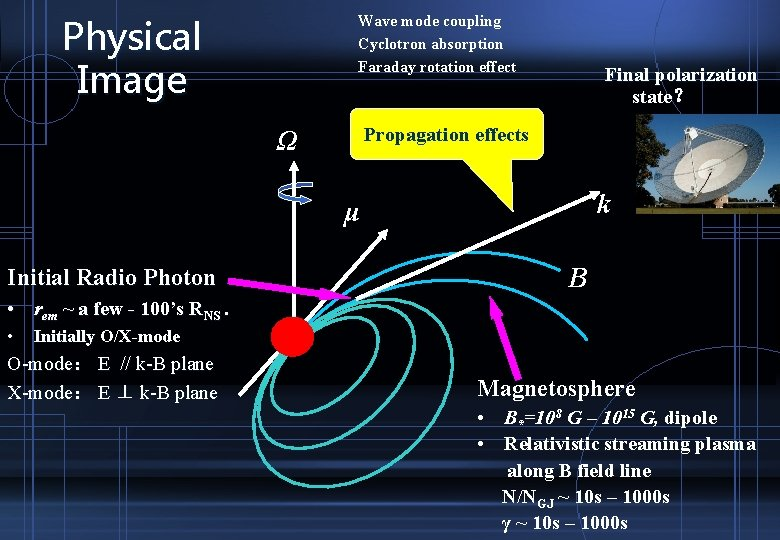Physical Image Wave mode coupling Cyclotron absorption Faraday rotation effect Final polarization state？ Propagation effects Ω k μ Initial Radio Photon B • rem ~ a few - 100’s RNS. • Initially O/X-mode O-mode： E // k-B plane X-mode： E ⊥ k-B plane Magnetosphere • B*=108 G – 1015 G, dipole • Relativistic streaming plasma along B field line N/NGJ ~ 10 s – 1000 s γ ~ 10 s – 1000 s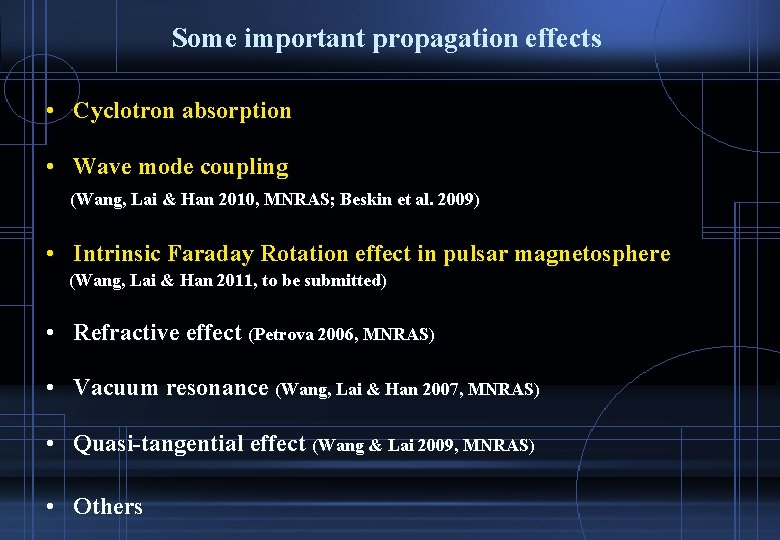Some important propagation effects • Cyclotron absorption • Wave mode coupling (Wang, Lai & Han 2010, MNRAS; Beskin et al. 2009) • Intrinsic Faraday Rotation effect in pulsar magnetosphere (Wang, Lai & Han 2011, to be submitted) • Refractive effect (Petrova 2006, MNRAS) • Vacuum resonance (Wang, Lai & Han 2007, MNRAS) • Quasi-tangential effect (Wang & Lai 2009, MNRAS) • Others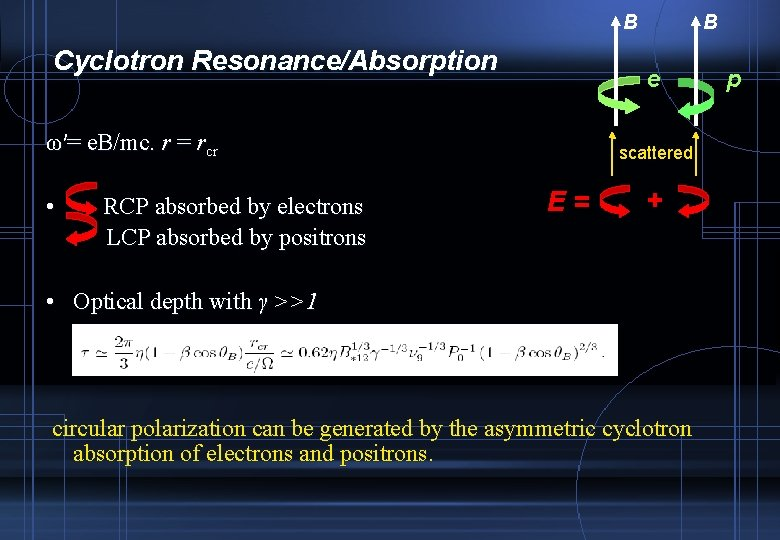B Cyclotron Resonance/Absorption e ω′= e. B/mc. r = rcr • RCP absorbed by electrons LCP absorbed by positrons B scattered E= + • Optical depth with γ >>1 circular polarization can be generated by the asymmetric cyclotron absorption of electrons and positrons. pWave Mode Coupling • The evolution of two linear eigenmodes from adiabatic to nonadiabatic. • rpl - polarization limiting radius, defined by – r << rpl , adiabatic mode evolution – r >> rpl , non-adiabatic mode evolution • Before WMC, PA follows the B field line plane After WMC, the polarization states are frozen • Circular polarization generated by wave mode coupling.Single Photon evolution along the ray Cyclotron absorption CP generated by wave mode couplingAn Interesting Application for WMC • Conal-double pulsars, PA increase V < 0 PA decrease V > 0 Can be explained easily by wave mode coupling effect CP generated by Wave mode coupling:Intrinsic Faraday Rotation in pulsar magnetosphere • Faraday rotation effect : two natural circular polarized modes have different phase velocities. Δk =Δnω/c • FR of Pulsars in ISM (non-relativistic electrons, B~u. G) is used to measure interstellar B field. • RM = RM_ISM + RM_PSR • Pulsar Magnetosphere – Strong B field – relativistic streaming plasma – Natural modes are linear polarized in inner magnetosphere and circularly polarized in outer magnetosphere –Δk no longer prop. to λ^2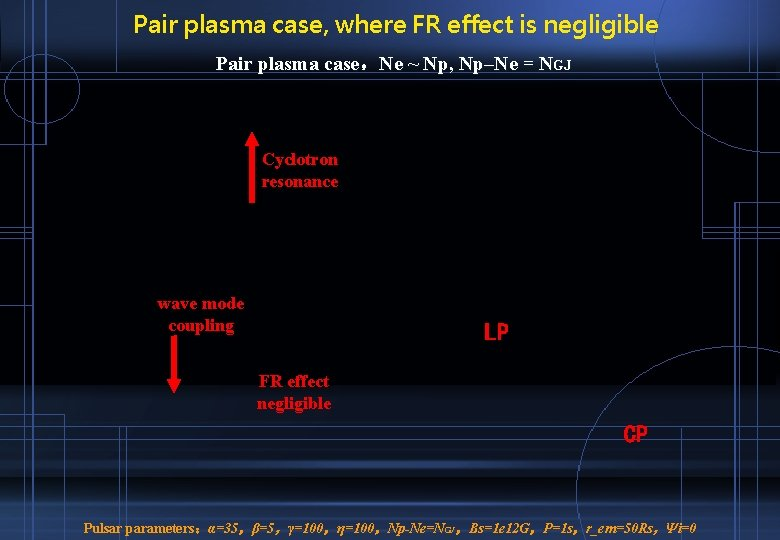Pair plasma case, where FR effect is negligible Pair plasma case，Ne ~ Np, Np–Ne = NGJ Cyclotron resonance wave mode coupling LP FR effect negligible CP Pulsar parameters：α=35，β=5，γ=100，η=100，Np-Ne=NGJ，Bs=1 e 12 G，P=1 s，r_em=50 Rs，Ψi=0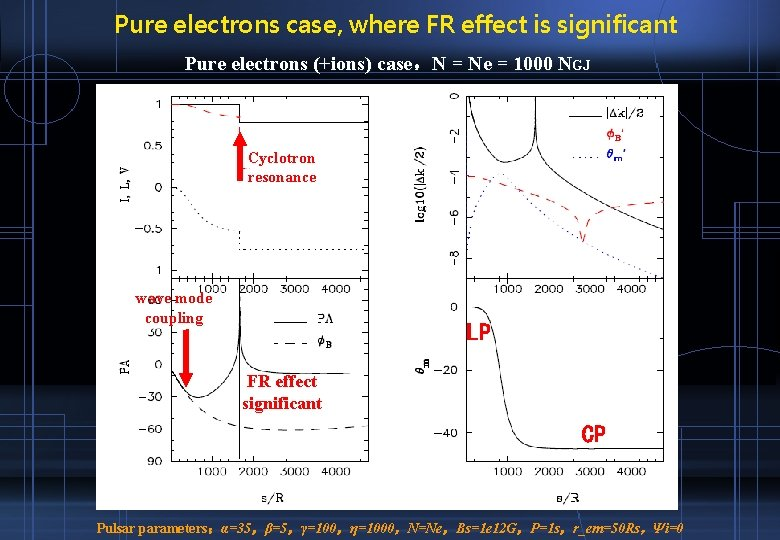Pure electrons case, where FR effect is significant Pure electrons (+ions) case，N = Ne = 1000 NGJ Cyclotron resonance wave mode coupling LP FR effect significant CP Pulsar parameters：α=35，β=5，γ=100，η=1000，N=Ne，Bs=1 e 12 G，P=1 s，r_em=50 Rs，Ψi=0Rotation Measure for the assumed pure electrons case • Subtract the influence to PA from other propagation effects • RM defined by Pulsar parameters：α=35，β=5，γ=100，η=1000，Bs=1 e 12 G，P=1 s，r_em=50 Rs，Ψi=0Conclusion • The propagation effects in pulsar magnetospheres could modify both the intensity and the polarization states of the emission from inner magnetosphere • CP can be generated in wave mode coupling, which maybe the reason of the relation between PA profile and the sign of CP in Conal-Double pulsars. • For symmetric pair plasma case (e. g. Goldreich-Julian model), intrinsic Faraday rotation in pulsar magnetosphere is negligible • Only for the assumed highly asymmetric plasma (e. g. , a electrons-ions streams with Ne >> NGJ) , FR maybe significant. FR angle is proportional to λ^~0. 5, not 2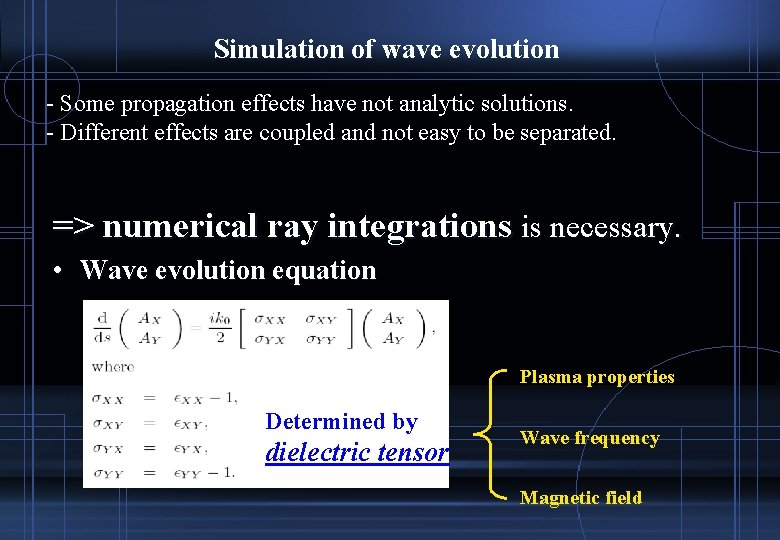Simulation of wave evolution - Some propagation effects have not analytic solutions. - Different effects are coupled and not easy to be separated. => numerical ray integrations is necessary. • Wave evolution equation Plasma properties Determined by dielectric tensor Wave frequency Magnetic fieldk k μ aligned μ k μ inversely aligned μ kPA evolution along the way for different parameters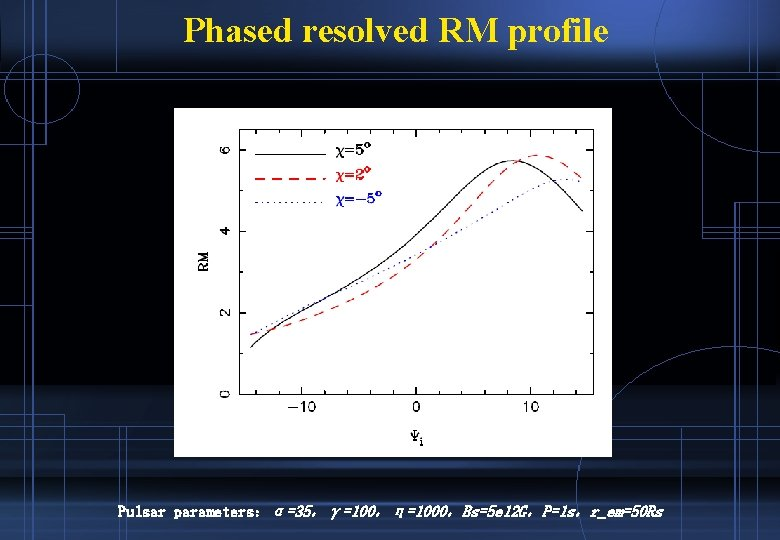Phased resolved RM profile Pulsar parameters：α=35，γ=100，η=1000，Bs=5 e 12 G，P=1 s，r_em=50 Rs